# XIRR

Calculates the internal rate of return of an investment based on a specified series of potentially irregularly spaced cash flows.

### Sample Usage

`XIRR(B2:B25,C2:C25)`

`XIRR({-4000,200,250,300},{DATE(2012,01,01),DATE(2012,06,23),DATE(2013,05,12),DATE(2014,02,09)},0.09)`

### Syntax

`XIRR(cashflow_amounts, cashflow_dates, [rate_guess])`

• `cashflow_amounts` - An array or range containing the income or payments associated with the investment.

• `cashflow_amounts` must contain at least one negative and one positive cash flow to calculate rate of return.
• `cashflow_dates` - An array or range with dates corresponding to the cash flows in `cashflow_amounts`.

• `rate_guess` - [ OPTIONAL - 0.1 by default ] - An estimate for what the internal rate of return will be.

### Notes

• If the days specified in `cashflow_dates` are at a regular interval, use `IRR` instead.

• Each cell in `cashflow_amounts` should be positive if it represents income from the perspective of the owner of the investment (e.g. coupons) or negative if it represents payments (e.g. loan repayment).

• `XNPV` will return zero if `discount` is set to the result of `XIRR` using the same cash flow amounts and schedule.

`XNPV`: Calculates the net present value of an investment based on a specified series of potentially irregularly spaced cash flows and a discount rate.

`PV`: Calculates the present value of an annuity investment based on constant-amount periodic payments and a constant interest rate.

`NPV`: Calculates the net present value of an investment based on a series of periodic cash flows and a discount rate.

`MIRR`: Calculates the modified internal rate of return on an investment based on a series of periodic cash flows and the difference between the interest rate paid on financing versus the return received on reinvested income.

`IRR`: Calculates the internal rate of return on an investment based on a series of periodic cash flows.

# TBILLYIELD

Calculates the yield of a US Treasury Bill based on price.

### Sample Usage

`TBILLYIELD(DATE(2010,1,2), DATE(2010,12,31), 98.45)`

`TBILLYIELD(A2,B2,C2)`

### Syntax

`TBILLYIELD(settlement, maturity, price)`

• `settlement` - The settlement date of the security, the date after issuance when the security is delivered to the buyer.

• `maturity` - The maturity or end date of the security, when it can be redeemed at face or par value.

• `price` - The price at which the security is bought.

### Notes

• `TBILLYIELD` requires the maturity date to be no more than a year after the settlement date.

• `settlement` and `maturity` should be entered using `DATE`, `TO_DATE` or other date parsing functions rather than by entering text.

• `TBILLYIELD` is equivalent to using `YIELDDISC` with US Treasury Bill conventions for the absent parameters.

`YIELDDISC`: Calculates the annual yield of a discount (non-interest-bearing) security, based on price.

`YIELD`: Calculates the annual yield of a security paying periodic interest, such as a US Treasury Bond, based on price.

# SYD

The SYD function calculates the depreciation of an asset for a specified period using the sum of years digits method.

### Sample Usage

`SYD(100,50,10,2)`

`SYD(A2,A3,A4,A5)`

### Syntax

`SYD(cost, salvage, life, period)`

• `cost` - The initial cost of the asset.

• `salvage` - The value of the asset at the end of depreciation.

• `life` - The number of periods over which the asset is depreciated.

• `period` - The single period within `life` for which to calculate depreciation.

### Notes

• `life` and `period` must be measured in the same units.

`SLN`: The SLN function calculates the depreciation of an asset for one period using the straight-line method.

`DDB`: The DDB function calculates the depreciation of an asset for a specified period using the double-declining balance method.

`DB`: The DB function calculates the depreciation of an asset for a specified period using the arithmetic declining balance method.

# RATE

Calculates the interest rate of an annuity investment based on constant-amount periodic payments and the assumption of a constant interest rate.

### Sample Usage

`RATE(12,-100,400,0,0,0.1)`

`RATE(A2,B2,C2,D2,1,0.08)`

### Syntax

`RATE(number_of_periods, payment_per_period, present_value, [future_value, end_or_beginning, rate_guess])`

• `number_of_periods` - The number of payments to be made.

• `payment_per_period` - The amount per period to be paid.

• `present_value` - The current value of the annuity.

• `future_value` - [ OPTIONAL ] - The future value remaining after the final payment has been made.

• `end_or_beginning` - [ OPTIONAL - `0` by default ] - Whether payments are due at the end (`0`) or beginning (`1`) of each period.

• `rate_guess` - [ OPTIONAL - 0.1 by default ] - An estimate for what the interest rate will be.

`PV`: Calculates the present value of an annuity investment based on constant-amount periodic payments and a constant interest rate.

`PPMT`: The PPMT function calculates the payment on the principal of an investment based on constant-amount periodic payments and a constant interest rate.

`PMT`: The PMT function calculates the periodic payment for an annuity investment based on constant-amount periodic payments and a constant interest rate.

`NPER`: The NPER function calculates the number of payment periods for an investment based on constant-amount periodic payments and a constant interest rate.

`IPMT`: The IPMT function calculates the payment on interest for an investment based on constant-amount periodic payments and a constant interest rate.

`FVSCHEDULE`: The FVSCHEDULE function calculates the future value of some principal based on a specified series of potentially varying interest rates.

`FV`: The FV function calculates the future value of an annuity investment based on constant-amount periodic payments and a constant interest rate.

`CUMPRINC`: Calculates the cumulative principal paid over a range of payment periods for an investment based on constant-amount periodic payments and a constant interest rate.

`CUMIPMT`: Calculates the cumulative interest over a range of payment periods for an investment based on constant-amount periodic payments and a constant interest rate.

### Use QuickTake to capture video while taking photos - Apple Support

Use QuickTake to capture video while taking photos

With iOS 13 and later, you can use QuickTake to capture a video while taking photos — without switching modes.

You can use the QuickTake camera on these devices: iPhone XS, iPhone XR, and later.## Hold the shutter to take a video

When you open the Camera app, you see the default photo mode. Tap the Shutter button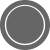to take a photo. Tap the arrow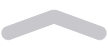to adjust options like flash, Live Photos, Night mode, timer, and more.

To capture a QuickTake video, just press and hold the Shutterbutton.* Release the button to stop recording.

On an iPhone XS, iPhone XR, and later with iOS 14, you can hold one of the Volume buttons to capture a QuickTake video. If you have Use Volume Up for Burst enabled, you can capture a QuickTake video with the Volume down button.

## Slide to the right to lock recording

To keep recording video without having to hold the button, slide the Shutterbutton to the right, then release it. When video recording is locked, a shutter button appears to the right; tap it to take a still photo during video recording. When you're ready to stop recording, tap the record button.

## Slide to the left for burst mode

Slide the Shutterbutton to the left and hold it to take a burst of photos, then release it to stop.

On an iPhone XS, iPhone XR, and later with iOS 14, you can capture photos in burst mode by pressing the Volume up button. Just go to Settings > Camera and turn on Use Volume Up for Burst.

* To take videos with customizable resolution, stereo audio, and audio zoom, switch to Video mode.Published Date:

# YIELD

Calculates the annual yield of a security paying periodic interest, such as a US Treasury Bond, based on price.

### Sample Usage

`YIELD(DATE(2010,01,02),DATE(2039,12,31),3,93.45,100,2)`

`YIELD(A2,B2,C2,D2,E2,F2,1)`

### Syntax

`YIELD(settlement, maturity, rate, price, redemption, frequency, [day_count_convention])`

• `settlement` - The settlement date of the security, the date after issuance when the security is delivered to the buyer.

• `maturity` - The maturity or end date of the security, when it can be redeemed at face, or par value.

• `rate` - The annualized rate of interest.

• `price` - The price at which the security is bought per 100 face value.

• `redemption` - The redemption amount per 100 face value, or par.

• `frequency` - The number of interest or coupon payments per year (1, 2, or 4).

• `day_count_convention` - [ OPTIONAL - `0` by default ] - An indicator of what day count method to use.

• 0 indicates US (NASD) 30/360 - This assumes 30 day months and 360 day years as per the National Association of Securities Dealers standard, and performs specific adjustments to entered dates which fall at the end of months.

• 1 indicates Actual/Actual - This calculates based upon the actual number of days between the specified dates, and the actual number of days in the intervening years. Used for US Treasury Bonds and Bills, but also the most relevant for non-financial use.

• 2 indicates Actual/360 - This calculates based on the actual number of days between the specified dates, but assumes a 360 day year.

• 3 indicates Actual/365 - This calculates based on the actual number of days between the specified dates, but assumes a 365 day year.

• 4 indicates European 30/360 - Similar to `0`, this calculates based on a 30 day month and 360 day year, but adjusts end-of-month dates according to European financial conventions.

### Notes

• `settlement` and `maturity` should be entered using `DATE`, `TO_DATE` or other date parsing functions rather than by entering text.

`YIELDDISC`: Calculates the annual yield of a discount (non-interest-bearing) security, based on price.

`TBILLYIELD`: Calculates the yield of a US Treasury Bill based on price.

`PRICEMAT`: Calculates the price of a security paying interest at maturity, based on expected yield.

`PRICEDISC`: Calculates the price of a discount (non-interest-bearing) security, based on expected yield.

`PRICE`: Calculates the price of a security paying periodic interest, such as a US Treasury Bond, based on expected yield.

`DISC`: Calculates the discount rate of a security based on price.

# TBILLPRICE

Calculates the price of a US Treasury Bill based on discount rate.

### Sample Usage

`TBILLPRICE(DATE(2010,1,2), DATE(2010,12,31), .0125)`

`TBILLPRICE(A2,B2,C2)`

### Syntax

`TBILLPRICE(settlement, maturity, discount)`

• `settlement` - The settlement date of the security, the date after issuance when the security is delivered to the buyer.

• `maturity` - The maturity or end date of the security, when it can be redeemed at face or par value.

• `discount` - The discount rate of the bill at time of purchase.

### Notes

• `settlement` and `maturity` should be entered using `DATE`, `TO_DATE` or other date parsing functions rather than by entering text.

• `TBILLPRICE` is equivalent to using `PRICEDISC` with US Treasury Bill conventions for the absent parameters.

• `maturity` must be one year or less from the `settlement` date.

• `discount` is a percentage and must be entered as a positive number from zero to one.

`TBILLYIELD`: Calculates the yield of a US Treasury Bill based on price.

`PRICEDISC`: Calculates the price of a discount (non-interest-bearing) security, based on expected yield.

`PRICE`: Calculates the price of a security paying periodic interest, such as a US Treasury Bond, based on expected yield.

# TBILLEQ

Calculates the equivalent annualized rate of return of a US Treasury Bill based on discount rate.

### Sample Usage

`TBILLEQ(DATE(2010,1,2), DATE(2010,12,31), .09)`

`TBILLEQ(A2,B2,C2)`

### Syntax

`TBILLEQ(settlement, maturity, discount)`

• `settlement` - The settlement date of the security, the date after issuance when the security is delivered to the buyer.

• `maturity` - The maturity or end date of the security, when it can be redeemed at face or par value.

• `discount` - The discount rate of the bill at time of purchase.

### Notes

• `settlement` and `maturity` should be entered using `DATE`, `TO_DATE` or other date parsing functions rather than by entering text.

`TBILLYIELD`: Calculates the yield of a US Treasury Bill based on price.

`TBILLPRICE`: Calculates the price of a US Treasury Bill based on discount rate.

# SLN

The SLN function calculates the depreciation of an asset for one period using the straight-line method.

### Sample Usage

`SLN(100,50,10)`

`SLN(A2,A3,A4)`

### Syntax

`SLN(cost, salvage, life)`

• `cost` - The initial cost of the asset.

• `salvage` - The value of the asset at the end of depreciation.

• `life` - The number of periods over which the asset is depreciated.

`SYD`: The SYD function calculates the depreciation of an asset for a specified period using the sum of years digits method.

`DDB`: The DDB function calculates the depreciation of an asset for a specified period using the double-declining balance method.

`DB`: The DB function calculates the depreciation of an asset for a specified period using the arithmetic declining balance method.

### How to move apps and create folders on your iPhone, iPad, or iPod touch - Apple Support

How to move apps and create folders on your iPhone, iPad, or iPod touch

You can organize the apps on your Home Screen, put them in folders, and move them to other pages, or screens. You can also reorder your pages.Touch and hold any app on the screen, then tap Edit Home Screen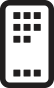.Now you can drag any app to another spot, including the Dock at the bottom of the screen.On iPhone X and later, tap Done to save. On iPhone 8 and earlier, press the Home button.## Put apps in a folder

If you have a lot of apps, you can put your related apps in folders.To make a folder, drag an app onto another app.If you want to rename the folder, tap the name field or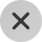and then enter the new name.Now that you have a folder, you can drag apps into it. Folders can have more than one page.## Move an app to a different page

You start with one page of apps. To make a new page, drag an app to the right edge of the screen. You might need to wait a second for the new page to appear. To delete a page, consolidate all your apps to another Home Screen. When there are no apps present the page will delete.

When you have more than one page, a bright dot in a row of dots above the Dock shows you which page you're on. You can move an app to a different page and create new pages:If there are dots to the left or right of the bright dot, you can drag an app to the right side of the screen to move the app to the next page in that direction. Or swipe left to see the apps on that page.If there are no dots to the right of the bright dot, dragging an app to that side of the screen makes a new page.Published Date:

### RECEIVED - Docs Editors Help [gg-docs-en]

Calculates the amount received at maturity for an investment in fixed-income securities purchased on a given date.

### Sample Usage

`RECEIVED(DATE(2010,02,01),DATE(2019,12,31),1000,0.05)`

`RECEIVED(A2,A3,A4,A5,1)`

### Syntax

`RECEIVED(settlement, maturity, investment, discount, [day_count_convention])`

• `settlement` - The settlement date of the security, the date after issuance when the security is delivered to the buyer.

• `maturity` - The maturity or end date of the security, when it can be redeemed at face or par value.

• `investment` - The amount invested (irrespective of face value of each security).

• `discount` - The discount rate of the security invested in.

• `day_count_convention` - [ OPTIONAL - `0` by default ] - An indicator of what day count method to use.

• 0 indicates US (NASD) 30/360 - This assumes 30 day months and 360 day years as per the National Association of Securities Dealers standard, and performs specific adjustments to entered dates which fall at the end of months.

• 1 indicates Actual/Actual - This calculates based upon the actual number of days between the specified dates, and the actual number of days in the intervening years. Used for US Treasury Bonds and Bills, but also the most relevant for non-financial use.

• 2 indicates Actual/360 - This calculates based on the actual number of days between the specified dates, but assumes a 360 day year.

• 3 indicates Actual/365 - This calculates based on the actual number of days between the specified dates, but assumes a 365 day year.

• 4 indicates European 30/360 - Similar to `0`, this calculates based on a 30 day month and 360 day year, but adjusts end-of-month dates according to European financial conventions.

### Notes

• `settlement` and `maturity` should be entered using `DATE`, `TO_DATE` or other date parsing functions rather than by entering text.

`YIELDDISC`: Calculates the annual yield of a discount (non-interest-bearing) security, based on price.

`YIELD`: Calculates the annual yield of a security paying periodic interest, such as a US Treasury Bond, based on price.

`PRICEMAT`: Calculates the price of a security paying interest at maturity, based on expected yield.

`PRICEDISC`: Calculates the price of a discount (non-interest-bearing) security, based on expected yield.

`PRICE`: Calculates the price of a security paying periodic interest, such as a US Treasury Bond, based on expected yield.

`MDURATION`: Calculates the modified Macaulay duration of a security paying periodic interest, such as a US Treasury Bond, based on expected yield.

`DURATION`: Calculates the number of compounding periods required for an investment of a specified present value appreciating at a given rate to reach a target value.

`DISC`: Calculates the discount rate of a security based on price.

`COUPPCD`: Calculates last coupon, or interest payment, date before the settlement date.

`COUPNCD`: Calculates next coupon, or interest payment, date after the settlement date.

`COUPDAYSNC`: Calculates the number of days from the settlement date until the next coupon, or interest payment.

`COUPDAYS`: Calculates the number of days in the coupon, or interest payment, period that contains the specified settlement date.

`COUPDAYBS`: Calculates the number of days from the first coupon, or interest payment, until settlement.

`ACCRINTM`: Calculates the accrued interest of a security that pays interest at maturity.

`ACCRINT`: Calculates the accrued interest of a security that has periodic payments.

# PV

Calculates the present value of an annuity investment based on constant-amount periodic payments and a constant interest rate.

### Sample Usage

`PV(2,12,100)`

`PV(A2,B2,C2,D2,1)`

### Syntax

`PV(rate, number_of_periods, payment_amount, [future_value], [end_or_beginning])`

• `rate` - The interest rate.

• `number_of_periods` - The number of payments to be made.

• `payment_amount` - The amount per period to be paid.

• `future_value` - [ OPTIONAL ] - The future value remaining after the final payment has been made.

• `end_or_beginning` - [ OPTIONAL - `0` by default ] - Whether payments are due at the end (`0`) or beginning (`1`) of each period.

### Notes

• Ensure that consistent units are used for `rate`, `number_of_periods`, and `payment_amount​`. For example, a car loan for 36 months may be paid monthly, in which case the annual percentage rate should be divided by 12 and the number of payments is 36. On the other hand, a different type of loan of the same length might be paid quarterly, in which case the annual percentage rate should be divided by 4 and the number of payments would be 12.

`PPMT`: The PPMT function calculates the payment on the principal of an investment based on constant-amount periodic payments and a constant interest rate.

`PMT`: The PMT function calculates the periodic payment for an annuity investment based on constant-amount periodic payments and a constant interest rate.

`NPER`: The NPER function calculates the number of payment periods for an investment based on constant-amount periodic payments and a constant interest rate.

`IPMT`: The IPMT function calculates the payment on interest for an investment based on constant-amount periodic payments and a constant interest rate.

`FVSCHEDULE`: The FVSCHEDULE function calculates the future value of some principal based on a specified series of potentially varying interest rates.

`FV`: The FV function calculates the future value of an annuity investment based on constant-amount periodic payments and a constant interest rate.

# PRICEDISC

Calculates the price of a discount (non-interest-bearing) security, based on expected yield.

### Sample Usage

`PRICEDISC(DATE(2010,01,02),DATE(2039,12,31),3,100)`

`PRICEDISC(A2,B2,C2,D2,1)`

### Syntax

`PRICEDISC(settlement, maturity, discount, redemption, [day_count_convention])`

• `settlement` - The settlement date of the security, the date after issuance when the security is delivered to the buyer.

• `maturity` - The maturity or end date of the security, when it can be redeemed at face or par value.

• `discount` - The discount rate of the security at time of purchase.

• `redemption` - The redemption value of the security.

• `day_count_convention` - [ OPTIONAL - `0` by default ] - An indicator of what day count method to use.

• 0 indicates US (NASD) 30/360 - This assumes 30 day months and 360 day years as per the National Association of Securities Dealers standard, and performs specific adjustments to entered dates which fall at the end of months.

• 1 indicates Actual/Actual - This calculates based upon the actual number of days between the specified dates, and the actual number of days in the intervening years. Used for US Treasury Bonds and Bills, but also the most relevant for non-financial use.

• 2 indicates Actual/360 - This calculates based on the actual number of days between the specified dates, but assumes a 360 day year.

• 3 indicates Actual/365 - This calculates based on the actual number of days between the specified dates, but assumes a 365 day year.

• 4 indicates European 30/360 - Similar to `0`, this calculates based on a 30 day month and 360 day year, but adjusts end-of-month dates according to European financial conventions.

### Notes

• `settlement` and `maturity` should be entered using `DATE`, `TO_DATE` or other date parsing functions rather than by entering text.

`YIELDDISC`: Calculates the annual yield of a discount (non-interest-bearing) security, based on price.

`YIELD`: Calculates the annual yield of a security paying periodic interest, such as a US Treasury Bond, based on price.

`TBILLYIELD`: Calculates the yield of a US Treasury Bill based on price.

`TBILLPRICE`: Calculates the price of a US Treasury Bill based on discount rate.

`TBILLEQ`: Calculates the equivalent annualized rate of return of a US Treasury Bill based on discount rate.

`PRICEMAT`: Calculates the price of a security paying interest at maturity, based on expected yield.

`PRICEDISC`: Calculates the price of a discount (non-interest-bearing) security, based on expected yield.

`DISC`: Calculates the discount rate of a security based on price.

# NOMINAL

Calculates the annual nominal interest rate given the effective rate and number of compounding periods per year.

### Sample Usage

`NOMINAL(0.85,12)`

`NOMINAL(A2,A3)`

### Syntax

`NOMINAL(effective_rate, periods_per_year)`

• `effective_rate` - The effective interest rate per year.

• `periods_per_year` - The number of compounding periods per year.

`INTRATE`: Calculates the effective interest rate generated when an investment is purchased at one price and sold at another with no interest or dividends generated by the investment itself.

`EFFECT`: Calculates the annual effective interest rate given the nominal rate and number of compounding periods per year.

### Delete apps on your iPhone, iPad, and iPod touch - Apple Support

Learn how to delete apps on your iPhone, iPad, and iPod touch.

## How to delete an app

1. Touch and hold the app.
2. Tap Remove App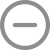.3. Tap Delete App, then tap Delete to confirm.

Keep in mind that some built-in Apple apps can't be deleted from your device, so you might not see the option to delete an app. Here's a list of built-in Apple apps you can delete from your device. If you can't delete an app that's not made by Apple, disable parental controls, then try deleting the app again.

If you touch and hold an app from the Home Screen and the apps start to jiggle:

1. Tap the Remove iconin the upper-left corner of the app.2. Tap Delete App, then tap Delete to confirm.
3. Tap Done.

• If you delete an app, it doesn't cancel in-app subscriptions that you might have. While deleting an app, you might see a Cancel Subscriptions option. Learn how to cancel your subscriptions.

Information about products not manufactured by Apple, or independent websites not controlled or tested by Apple, is provided without recommendation or endorsement. Apple assumes no responsibility with regard to the selection, performance, or use of third-party websites or products. Apple makes no representations regarding third-party website accuracy or reliability. Contact the vendor for additional information.

Published Date:

# IRR

Calculates the internal rate of return on an investment based on a series of periodic cash flows.

### Sample Usage

`IRR(A2:A25)`

`IRR({-4000,200,250,300,350},0.1)`

### Syntax

`IRR(cashflow_amounts, [rate_guess])`

• `cashflow_amounts` - An array or range containing the income or payments associated with the investment.

• `cashflow_amounts` must contain at least one negative and one positive cash flow to calculate rate of return.
• `rate_guess` - [ OPTIONAL - 0.1 by default ] - An estimate for what the internal rate of return will be.

### Notes

• Each cell in `cashflow_amounts` should be positive if it represents income from the perspective of the owner of the investment (e.g. coupons) or negative if it represents payments (e.g. loan repayment).

• `NPV` will return zero if `discount` is set to the result of `IRR` using the same cash flow amounts.

• If the cash flows of an investment are irregularly spaced, use `XIRR` instead.

`XNPV`: Calculates the net present value of an investment based on a specified series of potentially irregularly spaced cash flows and a discount rate.

`XIRR`: Calculates the internal rate of return of an investment based on a specified series of potentially irregularly spaced cash flows.

`PV`: Calculates the present value of an annuity investment based on constant-amount periodic payments and a constant interest rate.

`NPV`: Calculates the net present value of an investment based on a series of periodic cash flows and a discount rate.

`MIRR`: Calculates the modified internal rate of return on an investment based on a series of periodic cash flows and the difference between the interest rate paid on financing versus the return received on reinvested income.

# FVSCHEDULE

The FVSCHEDULE function calculates the future value of some principal based on a specified series of potentially varying interest rates.

### Sample Usage

`FVSCHEDULE(10000,A2:A100)`

`FVSCHEDULE(10000,{0.1,0.95,0.9,0.85})`

`FVSCHEDULE(A2,B2:B20)`

### Syntax

`FVSCHEDULE(principal, rate_schedule)`

• `principal` - The amount of initial capital or value to compound against.

• `rate_schedule` - A series of interest rates to compound against the `principal`.

• `rate_schedule` must be either a range or array containing the interest rates to compound, in sequence. These should be expressed either as decimals or as percentages using `UNARY_PERCENT`, i.e. `0.09` or `UNARY_PERCENT(9)` rather than `9`.

`PV`: Calculates the present value of an annuity investment based on constant-amount periodic payments and a constant interest rate.

`PPMT`: The PPMT function calculates the payment on the principal of an investment based on constant-amount periodic payments and a constant interest rate.

`PMT`: The PMT function calculates the periodic payment for an annuity investment based on constant-amount periodic payments and a constant interest rate.

`NPER`: The NPER function calculates the number of payment periods for an investment based on constant-amount periodic payments and a constant interest rate.

`IPMT`: The IPMT function calculates the payment on interest for an investment based on constant-amount periodic payments and a constant interest rate.

`FV`: The FV function calculates the future value of an annuity investment based on constant-amount periodic payments and a constant interest rate.

# FV

The FV function calculates the future value of an annuity investment based on constant-amount periodic payments and a constant interest rate.

### Sample Usage

`FV(2,12,100,400)`

`FV(A2,B2,C2,D2,1)`

### Syntax

`FV(rate, number_of_periods, payment_amount, [present_value], [end_or_beginning])`

• `rate` - The interest rate.

• `number_of_periods` - The number of payments to be made.

• `payment_amount` - The amount per period to be paid.

• `present_value` - [ OPTIONAL - `0` by default ] - The current value of the annuity.

• `end_or_beginning` - [ OPTIONAL - `0` by default ] - Whether payments are due at the end (`0`) or beginning (`1`) of each period.

### Notes

• Ensure that consistent units are used for `rate`, `number_of_periods`, and `payment_amount`. For example, a car loan for 36 months may be paid monthly, in which case the annual percentage rate should be divided by 12 and the number of payments is 36. On the other hand, a different type of loan of the same length might be paid quarterly, in which case the annual percentage rate should be divided by 4 and the number of payments would be 12.

`PV`: Calculates the present value of an annuity investment based on constant-amount periodic payments and a constant interest rate.

`PPMT`: The PPMT function calculates the payment on the principal of an investment based on constant-amount periodic payments and a constant interest rate.

`PMT`: The PMT function calculates the periodic payment for an annuity investment based on constant-amount periodic payments and a constant interest rate.

`NPER`: The NPER function calculates the number of payment periods for an investment based on constant-amount periodic payments and a constant interest rate.

`IPMT`: The IPMT function calculates the payment on interest for an investment based on constant-amount periodic payments and a constant interest rate.

`FVSCHEDULE`: The FVSCHEDULE function calculates the future value of some principal based on a specified series of potentially varying interest rates.

# EFFECT

Calculates the annual effective interest rate given the nominal rate and number of compounding periods per year.

### Sample Usage

`EFFECT(0.99,12)`

`EFFECT(A2,A3)`

### Syntax

`EFFECT(nominal_rate, periods_per_year)`

• `nominal_rate` - The nominal interest rate per year.

• `periods_per_year` - The number of compounding periods per year.

`NOMINAL`: Calculates the annual nominal interest rate given the effective rate and number of compounding periods per year.

`INTRATE`: Calculates the effective interest rate generated when an investment is purchased at one price and sold at another with no interest or dividends generated by the investment itself.

## Before you begin

• iPad Air 2 or later

• iPad (5th generation) or later
• iPad mini 4 or later2. Make sure that your accessory is in pairing or discovery mode.
• Apple Magic Mouse and Magic Trackpad should be ready to pair at this point.
• If you're pairing a third-party accessory that you've used with another device, follow the pairing-mode steps from its manufacturer.
4. Tap Bluetooth.
5. Look under Other Devices for your Bluetooth accessory, then tap its name to pair it with your iPad.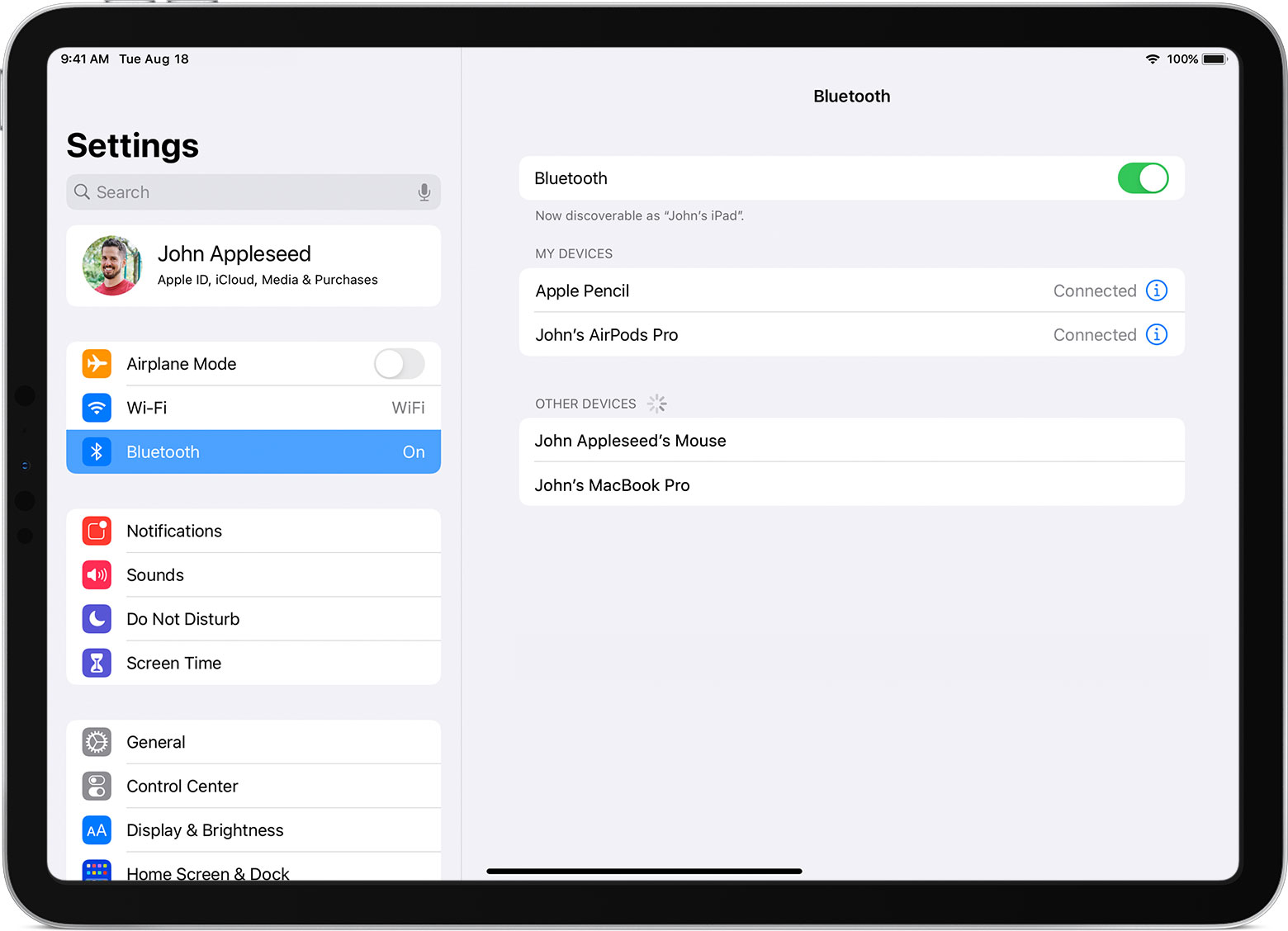Depending on the accessory that you're connecting, you might need to take extra steps. Follow any onscreen instructions that appear. If you're pairing with Apple Magic Mouse or Magic Trackpad and you're asked to provide a code, use 0000 (four zeros).

If you're having trouble connecting your accessory, try these tips.

iPadOS doesn't support scrolling or other gestures with Apple Magic Mouse (1st generation) or Magic Trackpad (1st generation).

## If you see a connectivity alert

When using a Bluetooth mouse or trackpad with your iPad, you might see an alert that says "Using this accessory may affect Wi-Fi and Bluetooth connectivity."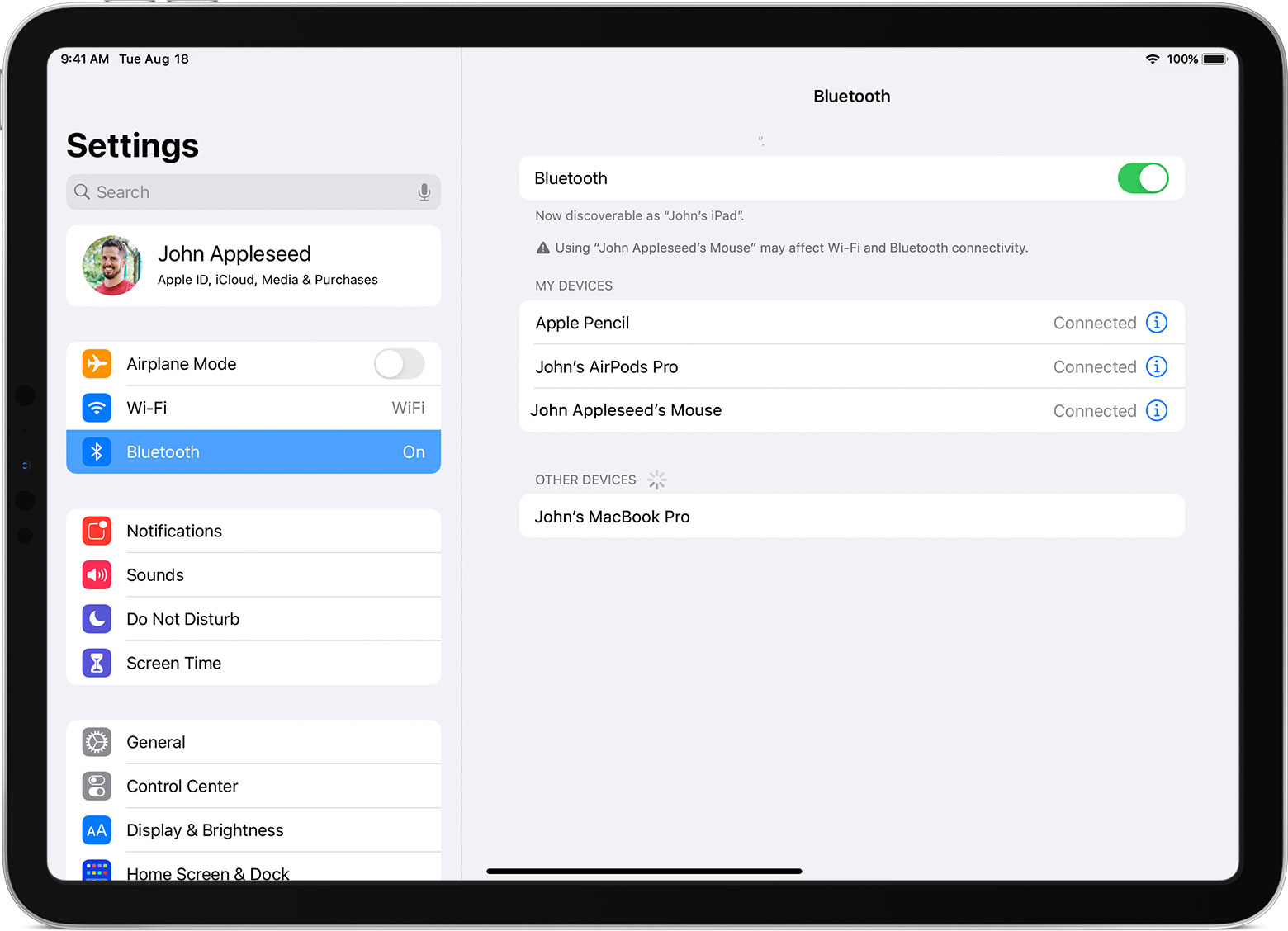Wireless interference can happen when you use a Bluetooth accessory with some iPad models. The more Bluetooth accessories that are connected to your iPad and active, the more likely you are to experience wireless performance issues.

If this happens, and you have connectivity issues, try disconnecting any Bluetooth accessories that you're not using. In Settings > Bluetooth, tap the information buttonnext to the accessory's name, then tap Disconnect.## Reconnect, disconnect, or forget the Bluetooth accessory

After it pairs with your iPad for the first time, your accessory appears under My Devices in Settings > Bluetooth. Tap your accessory's name to reconnect it to your iPad when it's not connected.

To disconnect the accessory or have your iPad forget the accessory, tap the information buttonnext to its name.• Using a wireless accessory with your iPad is similar to using one with a desktop or notebook computer. But there are some features that make the experience unique to iPad. Learn how to use a Bluetooth mouse or trackpad with your iPad.
• You can see the battery percentage of your connected Bluetooth mouse or trackpad in the Batteries widget. Learn how to add widgets in the Today View on your iPad.
• Don't see the onscreen keyboard when you have a trackpad or mouse connected? Tap the keyboard buttonin the lower-right corner of the screen. In the menu that appears, tap the show keyboard button. To hide the software keyboard, tap the dismiss button.

Information about products not manufactured by Apple, or independent websites not controlled or tested by Apple, is provided without recommendation or endorsement. Apple assumes no responsibility with regard to the selection, performance, or use of third-party websites or products. Apple makes no representations regarding third-party website accuracy or reliability. Contact the vendor for additional information.

Published Date:

# DISC

Calculates the discount rate of a security based on price.

### Sample Usage

`DISC(DATE(2010,01,02),DATE(2039,12,31),90,100)`

`DISC(A2,B2,C2,D2,1)`

### Syntax

`DISC(settlement, maturity, price, redemption, [day_count_convention])`

• `settlement` - The settlement date of the security, the date after issuance when the security is delivered to the buyer.

• `maturity` - The maturity or end date of the security, when it can be redeemed at face or par value.

• `price` - The price at which the security is bought.

• `redemption` - The redemption value of the security.

• `day_count_convention` - [ OPTIONAL - `0` by default ] - An indicator of what day count method to use.

• 0 indicates US (NASD) 30/360 - This assumes 30 day months and 360 day years as per the National Association of Securities Dealers standard, and performs specific adjustments to entered dates which fall at the end of months.

• 1 indicates Actual/Actual - This calculates based upon the actual number of days between the specified dates, and the actual number of days in the intervening years. Used for US Treasury Bonds and Bills, but also the most relevant for non-financial use.

• 2 indicates Actual/360 - This calculates based on the actual number of days between the specified dates, but assumes a 360 day year.

• 3 indicates Actual/365 - This calculates based on the actual number of days between the specified dates, but assumes a 365 day year.

• 4 indicates European 30/360 - Similar to `0`, this calculates based on a 30 day month and 360 day year, but adjusts end-of-month dates according to European financial conventions.

### Notes

• `settlement` and `maturity` should be entered using `DATE`, `TO_DATE` or other date parsing functions rather than by entering text.

`YIELDDISC`: Calculates the annual yield of a discount (non-interest-bearing) security, based on price.

`YIELD`: Calculates the annual yield of a security paying periodic interest, such as a US Treasury Bond, based on price.

`PRICEMAT`: Calculates the price of a security paying interest at maturity, based on expected yield.

`PRICEDISC`: Calculates the price of a discount (non-interest-bearing) security, based on expected yield.

`PRICE`: Calculates the price of a security paying periodic interest, such as a US Treasury Bond, based on expected yield.

# CUMIPMT

Calculates the cumulative interest over a range of payment periods for an investment based on constant-amount periodic payments and a constant interest rate.

### Sample Usage

`CUMIPMT(0.12,12,100,1,5,0)`

`CUMIPMT(A2,B2,C2,D2,E2,1)`

### Syntax

`CUMIPMT(rate, number_of_periods, present_value, first_period, last_period, end_or_beginning)`

• `rate` - The interest rate.

• `number_of_periods` - The number of payments to be made.

• `present_value` - The current value of the annuity.

• `first_period` - The number of the payment period to begin the cumulative calculation.

• `first_period` must be greater than or equal to `1`.
• `last_period` - The number of the payment period to end the cumulative calculation.

• `last_period` must be greater than `first_period`.
• `end_or_beginning` - Whether payments are due at the end (`0`) or beginning (`1`) of each period.

### Notes

• Ensure that consistent units are used for `rate` and `number_of_periods`. For example, a car loan for 36 months may be paid monthly, in which case the annual percentage rate should be divided by 12 and the number of payments is 36. On the other hand, a different type of loan of the same length might be paid quarterly, in which case the annual percentage rate should be divided by 4 and the number of payments would be 12.

`RATE`: Calculates the interest rate of an annuity investment based on constant-amount periodic payments and the assumption of a constant interest rate.

`PV`: Calculates the present value of an annuity investment based on constant-amount periodic payments and a constant interest rate.

`PMT`: The PMT function calculates the periodic payment for an annuity investment based on constant-amount periodic payments and a constant interest rate.

`NPER`: The NPER function calculates the number of payment periods for an investment based on constant-amount periodic payments and a constant interest rate.

`IPMT`: The IPMT function calculates the payment on interest for an investment based on constant-amount periodic payments and a constant interest rate.

`FVSCHEDULE`: The FVSCHEDULE function calculates the future value of some principal based on a specified series of potentially varying interest rates.

`FV`: The FV function calculates the future value of an annuity investment based on constant-amount periodic payments and a constant interest rate.

`CUMPRINC`: Calculates the cumulative principal paid over a range of payment periods for an investment based on constant-amount periodic payments and a constant interest rate.

# COUPPCD

Calculates last coupon, or interest payment, date before the settlement date.

### Sample Usage

`COUPPCD(DATE(2010,02,01),DATE(2019,12,31),4)`

`COUPPCD(A2,A3,A4,1)`

### Syntax

`COUPPCD(settlement, maturity, frequency, [day_count_convention])`

• `settlement` - The settlement date of the security, the date after issuance when the security is delivered to the buyer.

• `maturity` - The maturity or end date of the security, when it can be redeemed at face or par value.

• `frequency` - The number of interest or coupon payments per year (1, 2, or 4).

• `day_count_convention` - [ OPTIONAL - `0` by default ] - An indicator of what day count method to use.

• 0 indicates US (NASD) 30/360 - This assumes 30 day months and 360 day years as per the National Association of Securities Dealers standard, and performs specific adjustments to entered dates which fall at the end of months.

• 1 indicates Actual/Actual - This calculates based upon the actual number of days between the specified dates, and the actual number of days in the intervening years. Used for US Treasury Bonds and Bills, but also the most relevant for non-financial use.

• 2 indicates Actual/360 - This calculates based on the actual number of days between the specified dates, but assumes a 360 day year.

• 3 indicates Actual/365 - This calculates based on the actual number of days between the specified dates, but assumes a 365 day year.

• 4 indicates European 30/360 - Similar to `0`, this calculates based on a 30 day month and 360 day year, but adjusts end-of-month dates according to European financial conventions.

### Notes

• `settlement` and `maturity` should be entered using `DATE`, `TO_DATE` or other date parsing functions rather than by entering text.

`YIELDDISC`: Calculates the annual yield of a discount (non-interest-bearing) security, based on price.

`YIELD`: Calculates the annual yield of a security paying periodic interest, such as a US Treasury Bond, based on price.

`RECEIVED`: Calculates the amount received at maturity for an investment in fixed-income securities purchased on a given date.

`PRICEMAT`: Calculates the price of a security paying interest at maturity, based on expected yield.

`PRICEDISC`: Calculates the price of a discount (non-interest-bearing) security, based on expected yield.

`PRICE`: Calculates the price of a security paying periodic interest, such as a US Treasury Bond, based on expected yield.

`MDURATION`: Calculates the modified Macaulay duration of a security paying periodic interest, such as a US Treasury Bond, based on expected yield.

`DURATION`: Calculates the number of compounding periods required for an investment of a specified present value appreciating at a given rate to reach a target value.

`DISC`: Calculates the discount rate of a security based on price.

`COUPPCD`: Calculates last coupon, or interest payment, date before the settlement date.

`COUPNUM`: Calculates the number of coupons, or interest payments, between the settlement date and the maturity date of the investment.

`COUPDAYSNC`: Calculates the number of days from the settlement date until the next coupon, or interest payment.

`COUPDAYS`: Calculates the number of days in the coupon, or interest payment, period that contains the specified settlement date.

`COUPDAYBS`: Calculates the number of days from the first coupon, or interest payment, until settlement.

`ACCRINTM`: Calculates the accrued interest of a security that pays interest at maturity.

`ACCRINT`: Calculates the accrued interest of a security that has periodic payments.

### Use Multi-Touch gestures on your Mac - Apple Support

Use Multi-Touch gestures on your Mac

With a Multi-Touch trackpad or Magic Mouse, you can tap, swipe, pinch, or spread one or more fingers to perform useful actions.

For more information about these gestures, choose Apple menu  > System Preferences, then click Trackpad. You can turn a gesture off, change the type of gesture, and learn which gestures work with your Mac.Tap to click
Tap with one finger to click.Secondary click (right-click)
Click or tap with two fingers.Smart zoom
Double-tap with two fingers to zoom in and back out of a webpage or PDF.Scroll
Slide two fingers up or down to scroll.1Zoom in or out
Pinch with two fingers to zoom in or out.Rotate
Move two fingers around each other to rotate a photo or other item.Swipe between pages
Swipe left or right with two fingers to show the previous or next page.Swipe left from the right edge with two fingers to show Notification Center.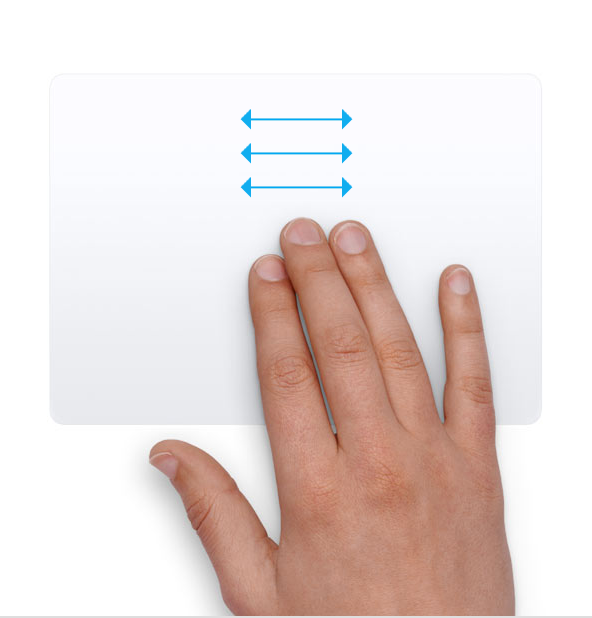Three finger drag
Use three fingers to drag items on your screen, then click or tap to drop. Turn on this feature in Accessibility preferences.Look up and data detectors
Tap with three fingers to look up a word or take actions with dates, addresses, phone numbers, and other data.Show desktopMission Control
Swipe up with four fingers2 to open Mission Control.App Exposé
Swipe down with four fingers2 to see all windows of the app you're using.Swipe between full-screen apps
Swipe left or right with four fingers2 to move between desktops and full-screen apps.## Mouse gestures

For more information about these gestures, choose Apple menu  > System Preferences, then click Mouse. There you can turn a gesture off, change the type of gesture, and learn which gestures work with your Mac. Mouse gestures require a Magic Mouse.Secondary click (right-click)
Click the right side of the mouse.Scroll
Slide one finger up or down to scroll.1Smart zoom
Double-tap with one finger to zoom in and back out of a webpage or PDF.Mission Control
Double-tap with two fingers to open Mission Control.Swipe between full-screen apps
Swipe left or right with two fingers to move between desktops and full-screen apps.Swipe between pages
Swipe left or right with one finger to show the previous or next page.1. You can turn off trackpad scrolling in Accessibility preferences.

2. In some versions of macOS, this gesture uses three fingers instead of four.

Published Date:

## Search This Blog

### Description of the InfoPath 2010 update 2817396: February 11, 2014 - Microsoft Support [MS]

Description of the InfoPath 2010 update 2817396: February 11, 2014 InfoP...